The LP Procedure
 TYPE Statement

TYPE variable ;

The TYPE statement specifies a character variable in the problem data set that contains the type identifier for each observation. This variable has keyword values that specify how the LP procedure should interpret the observation. If the TYPE statement is omitted, the procedure assumes that a variable named _TYPE_ contains the type keywords.

For the dense input format, the type variable identifies the constraint and objective rows and rows that contain information about the variables. The type variable should have nonmissing values in all observations.

For the sparse input format, the type variable identifies a model’s rows and columns. In an observation, a nonmissing type is associated with either a row or a column. If there are many columns sharing the same type, you can define a row of that type. Then, any nonmissing values in that row set the types of the corresponding columns.

The following are valid values for the TYPE variable in an observation:

 MIN contains the price coefficients of an objective row, for example,in the problem (MIP), to be minimized. MAX contains the price coefficients of an objective row, for example,, to be maximized. EQ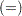contains coefficients of an equality constrained row. LE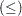contains coefficients of an inequality, less than or equal to, constrained row. GE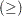contains coefficients of an inequality, greater than or equal to, constrained row. SOSEQ identifies the row as specifying a special ordered set. The variables flagged in this row are members of a set exactly one of which must be above its lower bound in the optimal solution. Note that variables in this type of special ordered set must be integer. SOSLE identifies the row as specifying a special ordered set. The variables flagged in this row are members of a set in which only one can be above its lower bound in the optimal solution. UNRSTRT UNRSTRCT identifies those structural variables to be considered as unrestricted variables. These are variables for which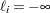and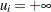. Any variable that has ain this observation is considered an unrestricted variable. LOWERBD identifies lower bounds on the structural variables. If all structural variables are to be nonnegative, that is,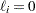, then you do not need to include an observation with the 'LOWERBD' keyword in a variable specified in the TYPE statement. Missing values for variables in a lower-bound row indicate that the variable has lower bound equal to zero. Note:A variable with lower or upper bounds cannot be identified as unrestricted. UPPERBD identifies upper bounds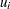on the structural variables. For each structural variable that is to have an upper bound, the observation must contain a missing value or the current value of INFINITY. All other values are interpreted as upper bounds, including 0. FIXED identifies variables that have fixed values. A nonmissing value in a row with 'FIXED' type keyword gives the constant value of that variable. INTEGER identifies variables that are integer-constrained. In a feasible solution, these variables must have integer values. A missing value in a row with 'INTEGER' type keyword indicates that the variable is not integer-constrained. The value of variables in the 'INTEGER' row gives an ordering to the integer-constrained variables that is used when the VARSELECT= option equals PRIOR. Note:Every integer-constrained variable must have an upper bound defined in a row with type 'UPPERBD'. See the section Controlling the Branch-and-Bound Search for further discussion. BINARY identifies variables that are constrained to be either 0 or 1. This is equivalent to specifying that the variable is an integer variable and has a lower bound of 0 and an upper bound of 1. A missing value in a row with 'BINARY' type keyword indicates that the variable is not constrained to be 0 or 1. The value of variables in the 'BINARY' row gives an ordering to the integer-constrained variables that is used when the VARSELECT= option equals PRIOR. See the section Controlling the Branch-and-Bound Search for further discussion. BASIC identifies variables that form an initial basic feasible solution. A missing value in a row with 'BASIC' type indicates that the variable is not basic. PRICESEN identifies a vector that is used to evaluate the sensitivity of the optimal solution to changes in the objective function. See the section Price Sensitivity Analysis and the section Price Parametric Programming for further discussion. FREE identifies a nonbinding constraint. Any number of FREE constraints can appear in a problem data set. RHS identifies a right-hand-side column in the sparse input format. This replaces the RHS statement. It is useful when converting the MPS format into the sparse format of PROC LP. See the section Converting Standard MPS Format to Sparse Format for more information. RHSSEN identifies a right-hand-side sensitivity analysis vector in the sparse input format. This replaces the RHSSEN statement. It is useful when converting the MPS format into the sparse format of PROC LP. See the section Converting Standard MPS Format to Sparse Format for more information. RANGE identifies a range vector in the sparse input format. This replaces the RANGE statement. It is useful when converting the MPS format into the sparse format of PROC LP. See the section Converting Standard MPS Format to Sparse Format for more information.Previous Page | Next Page | Top of Page